# Engineering Mechanics - Planar Kinematics of a Rigid Body (PKRB)

### Exercise :: Planar Kinematics of a Rigid Body (PKRB) - General Questions

• Planar Kinematics of a Rigid Body (PKRB) - General Questions
21.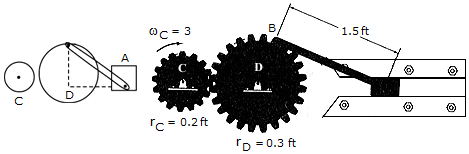Gear C is rotating with a constant angular velocity of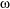c = 3 rad/s. Determine the acceleration of the piston A and the angular acceleration of rod AB at the instant= 90°. Set rc = 0.2 ft and rd = 0.3 ft.

 A. aA = 5.88 ft/s2 6, "AB = 4.00 rad/s2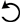B. aA = 5.88 ft/s2 7, "AB = 4.00 rad/s2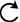C. aA = 0.245 ft/s2 6, "AB = 0.816 rad/s2D. aA = 0.245 ft/s2 7, "AB = 0.816 rad/s2Explanation:

No answer description available for this question. Let us discuss.

22.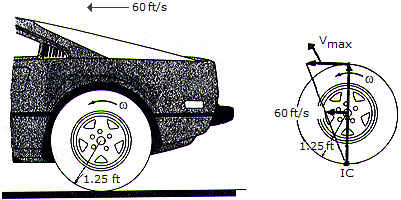The automobile with wheels 2.5 ft in diameter is traveling in a straight path at a rate of 60 ft/s. If no slipping occurs, determine the angular velocity of one of the rear wheels and the velocity of the fastest moving point on the wheel.

 A.= 48 rad/s, vmax = 84.9 ft/s B.= 24 rad/s, vmax = 84.9 ft/s C.= 48 rad/s, vmax = 120.0 ft/s D.= 24 rad/s, vmax = 120.0 ft/s

Explanation:

No answer description available for this question. Let us discuss.

23.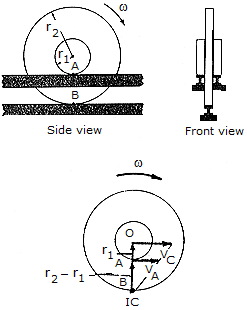If the rim of the wheel and its hub maintain contact with the three stationary tracks as the wheel rolls, it is neccessary that slipping occurs at the hub A if no slipping occurs at B. Under these conditions, what is the speed at A if the wheel has an angular velocity?

 A. vA = (r2-r1)B. vA = r1C. vA = (r2-r1)D. vA = r1